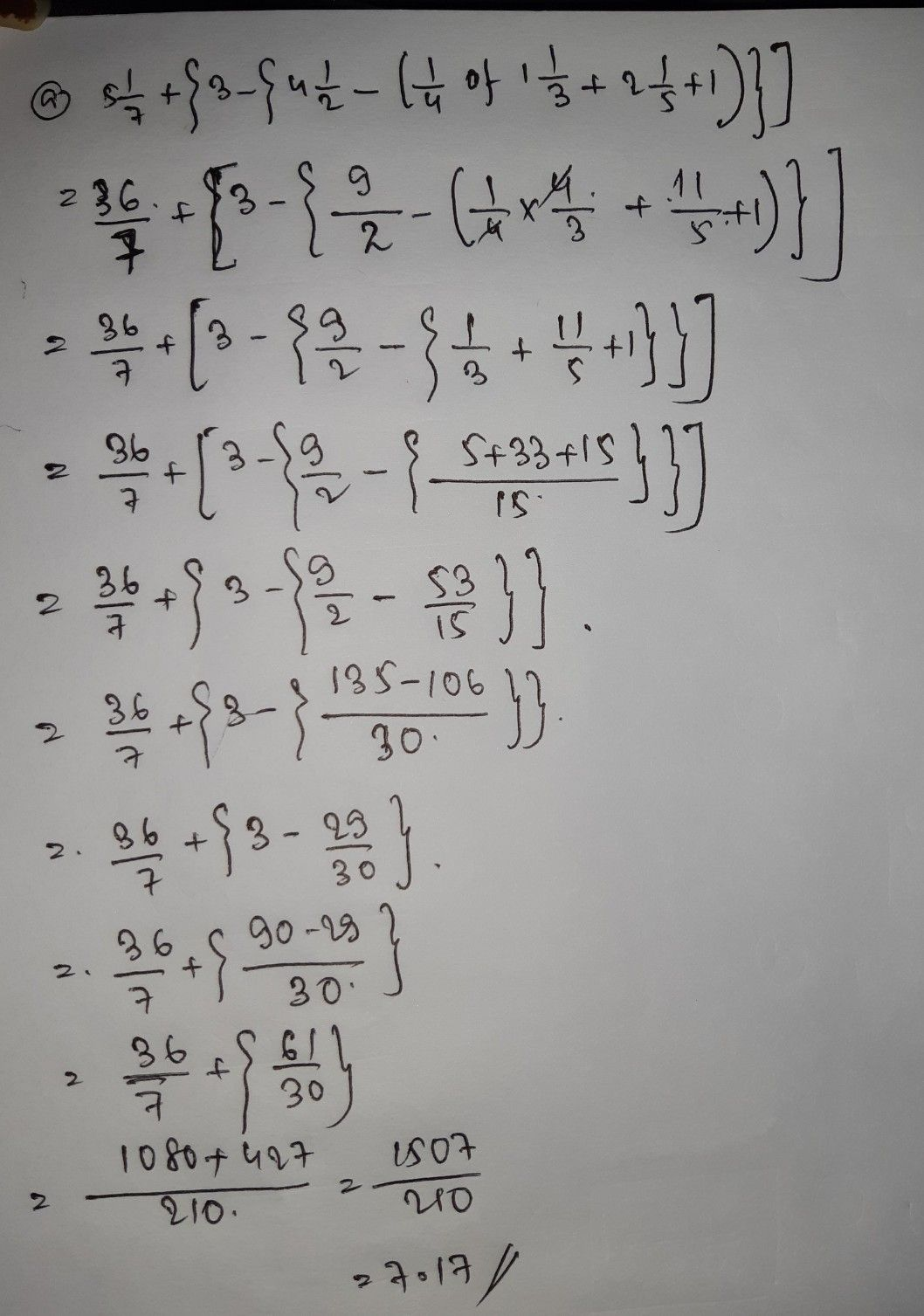Symbol
Problem2. Simplify the following expressions: a) st $+\left(3-$ {4} $-\left(\dfrac {1} {4}$ of 1 $4=2$ $+$ )}] c) 13 $-\left(\dfrac {1} {5}$ of (4 25 $+$ 3 of 5 $-6\dfrac {1} {4}\right)\right)$ Thinking There were 4 flavours of nizzas double cheese mocor
1st-6th grade
Other
SolutionQanda teacher - Shrestha